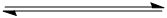# Chemical Equilibrium

Calculate the equilibrium concentration ratio of C to A if 2.0 ml of each of A and B were allowed to come to equilibrium at 300 K.
A + BC + D
dG = 460 cal

•Gaurav Gardi ·

K=[C][D][A][B]
we know that at equilibrium [C]=[D] and [A]=[B]
so k=[C]2[A]2
therefore [C][A]=âˆšK
also K=e-Î”GoRT
so,[C][A]=e-Î”Go2RT

•Hardik Sheth ·

i think the ans is the square root of the given ans by swarna..

Swarna Kamal Dhyawala ·

(deltaGÂ°)/-RT = lnK

K = e-deltaGÂ°/RT

Ans)1.8 x 10-4

Bitan Chakraborty ·

@Anurag and @Swarna Kamal: See the above solution. This is how you tackle sums related to equilibrium.
•